Next: Power and work Up: Rotational motion Previous: Moment of inertia

## Torque

We have now identified the rotational equivalent of velocity--namely, angular velocity--and the rotational equivalent of mass--namely, moment of inertia. But, what is the rotational equivalent of force?

Consider a bicycle wheel of radiuswhich is free to rotate around a perpendicular axis passing through its centre. Suppose that we apply a force, which is coplanar with the wheel, to a point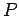lying on its circumference. See Fig. 79. What is the wheel's subsequent motion?

Let us choose the origin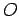of our coordinate system to coincide with the pivot point of the wheel--i.e., the point of intersection between the wheel and the axis of rotation. Letbe the position vector of point, and letbe the angle subtended between the directions ofand. We can resolveinto two components--namely, a component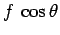which acts radially, and a component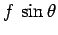which acts tangentially. The radial component ofis canceled out by a reaction at the pivot, since the wheel is assumed to be mounted in such a manner that it can only rotate, and is prevented from displacing sideways. The tangential component ofcauses the wheel to accelerate tangentially. Let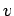be the instantaneous rotation velocity of the wheel's circumference. Newton's second law of motion, applied to the tangential motion of the wheel, yields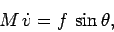(360)

where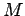is the mass of the wheel (which is assumed to be concentrated in the wheel's rim).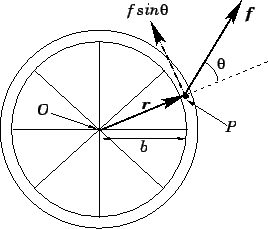Let us now convert the above expression into a rotational equation of motion. Ifis the instantaneous angular velocity of the wheel, then the relation betweenandis simply(361)

Since the wheel is basically a ring of radius, rotating about a perpendicular symmetric axis, its moment of inertia is(362)

Combining the previous three equations, we obtain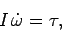(363)

where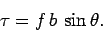(364)

Equation (363) is the angular equation of motion of the wheel. It relates the wheel's angular velocity,, and moment of inertia,, to a quantity,, which is known as the torque. Clearly, ifis analogous to mass, andis analogous to velocity, then torque must be analogous to force. In other words, torque is the rotational equivalent of force.

It is clear, from Eq. (364), that a torque is the product of the magnitude of the applied force,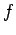, and some distance. The physical interpretation of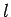is illustrated in Fig. 80. If can be seen thatis the perpendicular distance of the line of action of the force from the axis of rotation. We usually refer to this distance as the length of the lever arm.

In summary, a torque measures the propensity of a given force to cause the object upon which it acts to twist about a certain axis. The torque,, is simply the product of the magnitude of the applied force,, and the length of the lever arm,:(365)

Of course, this definition makes a lot of sense. We all know that it is far easier to turn a rusty bolt using a long, rather than a short, wrench. Assuming that we exert the same force on the end of each wrench, the torque we apply to the bolt is larger in the former case, since the perpendicular distance between the line of action of the force and the bolt (i.e., the length of the wrench) is greater.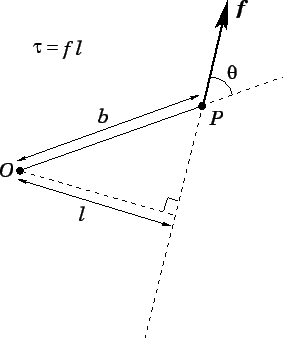Since force is a vector quantity, it stands to reason that torque must also be a vector quantity. It follows that Eq. (365) defines the magnitude,, of some torque vector,. But, what is the direction of this vector? By convention, if a torque is such as to cause the object upon which it acts to twist about a certain axis, then the direction of that torque runs along the direction of the axis in the sense given by the right-hand grip rule. In other words, if the fingers of the right-hand circulate around the axis of rotation in the sense in which the torque twists the object, then the thumb of the right-hand points along the axis in the direction of the torque. It follows that we can rewrite our rotational equation of motion, Eq. (363), in vector form:(366)

where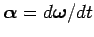is the vector angular acceleration. Note that the direction of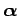indicates the direction of the rotation axis about which the object accelerates (in the sense given by the right-hand grip rule), whereas the direction ofindicates the direction of the rotation axis about which the torque attempts to twist the object (in the sense given by the right-hand grip rule). Of course, these two rotation axes are identical.

Although Eq. (366) was derived for the special case of a torque applied to a ring rotating about a perpendicular symmetric axis, it is, nevertheless, completely general.

It is important to appreciate that the directions we ascribe to angular velocities, angular accelerations, and torques are merely conventions. There is actually no physical motion in the direction of the angular velocity vector--in fact, all of the motion is in the plane perpendicular to this vector. Likewise, there is no physical acceleration in the direction of the angular acceleration vector--again, all of the acceleration is in the plane perpendicular to this vector. Finally, no physical forces act in the direction of the torque vector--in fact, all of the forces act in the plane perpendicular to this vector.

Consider a rigid body which is free to pivot in any direction about some fixed point. Suppose that a forceis applied to the body at some pointwhose position vector relative tois. See Fig. 81. Letbe the angle subtended between the directions ofand. What is the vector torqueacting on the object about an axis passing through the pivot point? The magnitude of this torque is simply(367)

In Fig. 81, the conventional direction of the torque is out of the page. Another way of saying this is that the direction of the torque is mutually perpendicular to bothand, in the sense given by the right-hand grip rule when vectoris rotated onto vector(through an angle less thandegrees). It follows that we can write(368)

In other words, the torque exerted by a force acting on a rigid body which pivots about some fixed point is the vector product of the displacement of the point of application of the force from the pivot point with the force itself. Equation (368) specifies both the magnitude of the torque, and the axis of rotation about which the torque twists the body upon which it acts. This axis runs parallel to the direction of, and passes through the pivot point.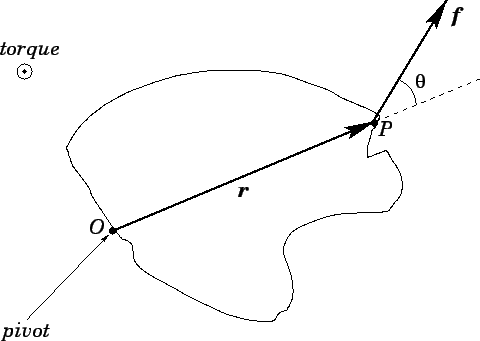Next: Power and work Up: Rotational motion Previous: Moment of inertia
Richard Fitzpatrick 2006-02-02